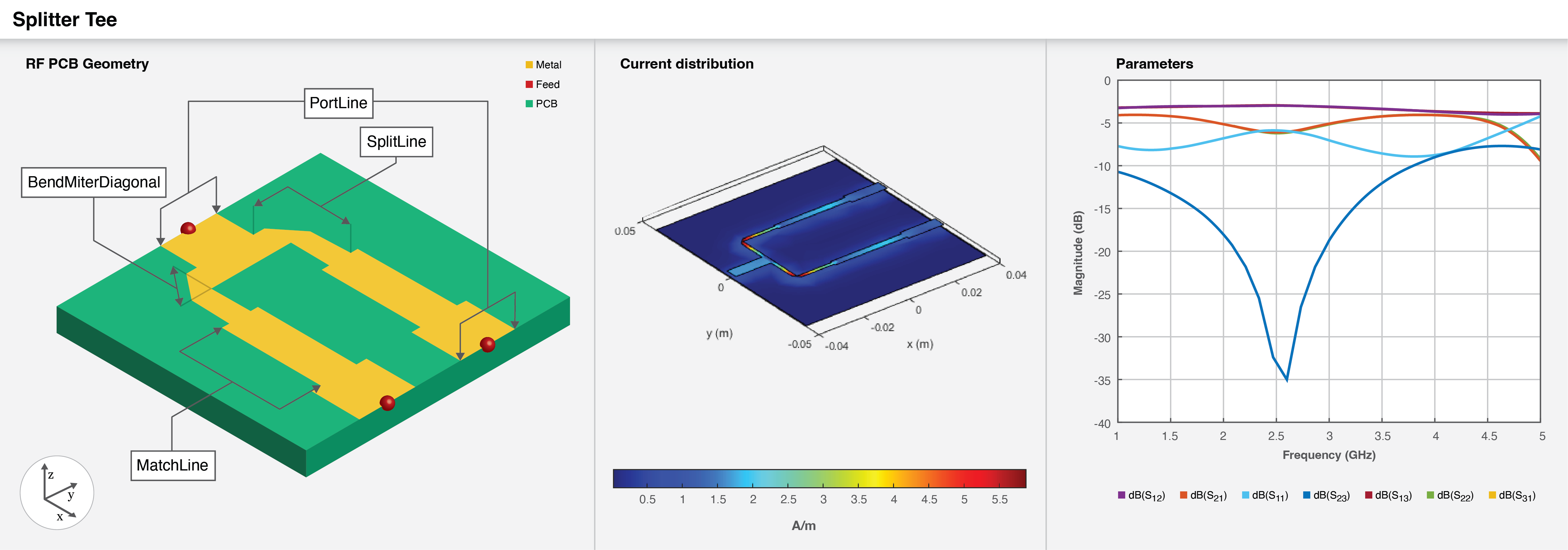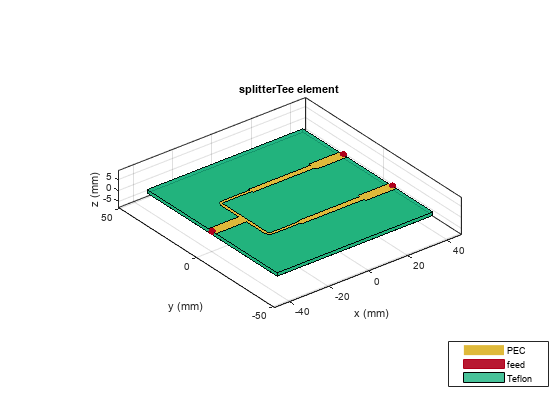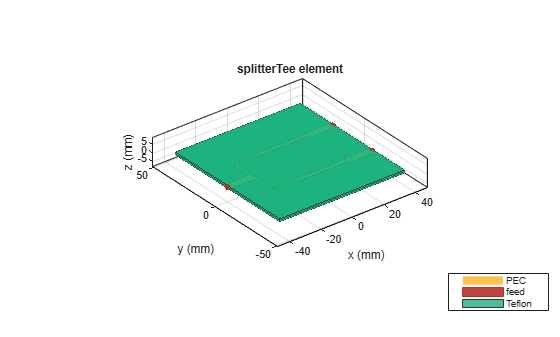# splitterTee

Create T-junction power splitter

Since R2022b

## Description

Use the `splitterTee` object to create a T-junction power splitter. The T-junction is a lossless and reciprocal three-port divider which divides the input equally between the two output ports.To analyze the behavioral model for the T-junction power splitter, set the `Behavioral` property in `sparameters` to `true` or `1`.

## Creation

### Syntax

``splitter = splitterTee``
``splitter = splitterTee(Name=Value)``

### Description

example

````splitter = splitterTee` creates a T-junction power splitter with default properties for a design frequency of 1.8 GHz.```

example

````splitter = splitterTee(Name=Value)` sets Properties using one or more name-value arguments. For example, `splitterTee(PortLineLength=0.0155)` creates a T-junction splitter with a port line length of 0.0155 meters. Properties not specified retain their default values.```

## Properties

expand all

Shape of the T-junction splitter, specified as `'RectangularMitered'`, `'RectangularCurved'`, or `'Circular'`.

Example: `splitter = splitterTee(Shape='Circular')`

Data Types: `char`

Length of the port line in meters, specified as a positive scalar.

Example: `splitter = splitterTee(PortLineLength=0.0144)`

Data Types: `double`

Width of the port line in meters, specified as a positive scalar.

Example: `splitter = splitterTee(PortLineWidth=0.0041)`

Data Types: `double`

Length of the split line in meters, specified as a positive scalar. Generally the length is `λ/4` for a Tee splitter.

Example: `splitter = splitterTee(SplitLineLength=0.0420)`

Data Types: `double`

Width of the split line in meters, specified as a positive scalar.

Example: `splitter = splitterTee(SplitLineWidth=0.0025)`

Data Types: `double`

Length of the miter diagonal at the bend in meters, specified as a positive scalar.

Example: `splitter = splitterTee(BendMiterDiagonal=0.0045)`

Data Types: `double`

Radius of the curve at the bend in meters, specified as a positive scalar.

Example: `splitter = splitterTee(BendCurveRadius=0.0030)`

#### Dependencies

To enable this property, set the `Shape` property to `'RectangularCurved'`.

Data Types: `double`

Length of the match line in meters, specified as a positive scalar.

Example: `splitter = splitterTee(MatchLineLength=0.0415)`

Data Types: `double`

Width of the match line in meters, specified as a positive scalar.

Example: `splitter = splitterTee(MatchLineWidth=0.0039)`

Data Types: `double`

Spacing between the output ports in meters, specified as a positive scalar.

Example: `splitter = splitterTee(PortSpacing=0.0420)`

Data Types: `double`

Height of the T-junction power splitter from the ground plane in meters, specified as a positive scalar.

In the case of a multilayer substrate, you can use the `Height` property to create a T-junction power splitter where the two dielectrics interface.

Example: `splitter = splitterTee(Height=0.0076)`

Data Types: `double`

Width of the ground plane in meters, specified as a positive scalar.

Example: `splitter = splitterTee(GroundPlaneWidth=0.098)`

Example: double

Type of dielectric material used as a substrate, specified as a `dielectric` object. The dielectric material in a `splitterTee` object with default properties in Teflon.

Example: `d = dielectric("FR4");` `splitter = splitterTee(Substrate=d)`

Data Types: `string` | `char`

Type of metal used in the conducting layers, specified as a `metal` object. The metal in a `splitterTee` object with default properties in PEC.

Example: `m = metal("Copper");` `splitter = splitterTee(Conductor=m)`

Data Types: `string` | `char`

## Object Functions

 `charge` Calculate and plot charge distribution `current` Calculate and plot current distribution `design` Design T-junction power splitter around specified frequency `feedCurrent` Calculate current at feed port `layout` Plot all metal layers and board shape `mesh` Change and view mesh properties of metal or dielectric in PCB component `shapes` Extract all metal layer shapes of PCB component `show` Display PCB component structure or PCB shape `sparameters` Calculate S-parameters for RF PCB objects

## Examples

collapse all

Create a default splitter tee.

`splitter = splitterTee`
```splitter = splitterTee with properties: Shape: 'RectangularMitered' PortLineLength: 0.0155 PortLineWidth: 0.0051 SplitLineLength: 0.0320 SplitLineWidth: 0.0015 BendMiterDiagonal: 0.0035 MatchLineLength: 0.0315 MatchLineWidth: 0.0029 PortSpacing: 0.0320 Height: 0.0016 GroundPlaneWidth: 0.0847 Substrate: [1x1 dielectric] Conductor: [1x1 metal] ```

Visualize the splitter tee.

`show(splitter)`Create a curved rectangular splitter tee.

`splitter = splitterTee(Shape='RectangularCurved')`
```splitter = splitterTee with properties: Shape: 'RectangularCurved' PortLineLength: 0.0155 PortLineWidth: 0.0051 SplitLineLength: 0.0320 SplitLineWidth: 0.0015 BendCurveRadius: 0.0020 MatchLineLength: 0.0315 MatchLineWidth: 0.0029 PortSpacing: 0.0320 Height: 0.0016 GroundPlaneWidth: 0.0847 Substrate: [1x1 dielectric] Conductor: [1x1 metal] ```

Visualize the splitter tee.

`show(splitter)`Calculate and plot the s-parameters of the tee at 3 GHz.

`spar = sparameters(splitter,3e9)`
```spar = sparameters: S-parameters object NumPorts: 3 Frequencies: 3.0000e+09 Parameters: [3x3 double] Impedance: 50 rfparam(obj,i,j) returns S-parameter Sij ```
`rfplot(spar)`Create a splitter tee at the interface of a multilayer dielectric.

```splitter = splitterTee; splitter.Substrate = dielectric(Name={'Teflon','Teflon'},EpsilonR=[2.1 2.1], ... LossTangent=[0 0],Thickness=[0.8e-3 0.8e-3]); splitter.Height = 0.8e-3;```

Visualize the splitter tee.

`show(splitter);`Pozar, David M. Microwave Engineering. 4th ed. Hoboken, NJ: Wiley, 2012.

 Kumari, Chanchala, and Neela Chattoraj. “Design of an Elementary Microstrip Power Splitter for Antenna Array.” In 2021 National Conference on Communications (NCC), 1–5. Kanpur, India: IEEE, 2021. https://doi.org/10.1109/NCC52529.2021.9530097.